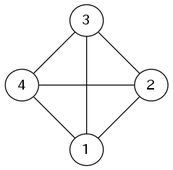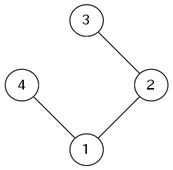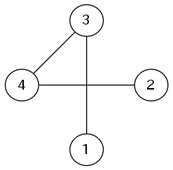시간 제한 메모리 제한 제출 정답 맞은 사람 정답 비율
2 초 512 MB 2 1 1 50.000%

## 문제

Consider a complete graph with $N$ vertices. Find $K$ spanning trees that are edge-disjoint.The leftmost figure above shows a complete graph with four vertices. The two figures to the right are two edge-disjoint spanning trees of this graph.

## 입력

You are given two integers $N$ and $K$ on a single line ($2 \le N \le 10^4$, $1 \le K \le 100$).

## 출력

If there is no tuple of $K$ spanning trees that satisfies the conditions, print $-1$.

Otherwise, print $K$ spanning trees. Each spanning tree must be printed on $N - 1$ lines. The $i$-th line must contain two space-separated integers: the two endpoints of the $i$-th edge. The vertices are numbered $1$ through $N$. You may print an empty line between consecutive trees.

## 예제 입력 1

4 2


## 예제 출력 1

1 2
1 4
2 3

1 3
2 4
3 4


## 예제 입력 2

4 3


## 예제 출력 2

-1